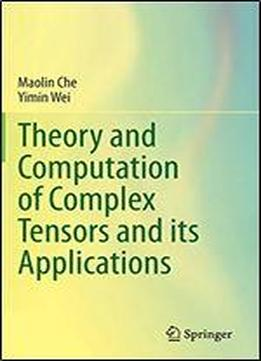# Theory And Computation Of Complex Tensors And Its Applications by Maolin Che / 2020 / English / PDF

The book provides an introduction of very recent results about the tensors and mainly focuses on the authors' work and perspective. A systematic description about how to extend the numerical linear algebra to the numerical multi-linear algebra is also delivered in this book. The authors design the neural network model for the computation of the rank-one approximation of real tensors, a normalization algorithm to convert some nonnegative tensors to plane stochastic tensors and a probabilistic algorithm for locating a positive diagonal in a nonnegative tensors, adaptive randomized algorithms for computing the approximate tensor decompositions, and the QR type method for computing U-eigenpairs of complex tensors. This book could be used for the Graduate course, such as Introduction to Tensor. Researchers may also find it helpful as a reference in tensor research.

views: 203